GeeksforGeeks App
Open AppBrowser
Continue

# Python Arcade – Collision Detection

## Installation

You can install the Arcade module using the below command:

`pip install arcade`

## Example 1:

In this example, we are going to use the check_for_collision() function to add collision between 2 sprites.

Parameters:

• sprite1:- First sprite
• sprite2:- Second sprite

Returns: Boolean value

#### Sprites Used: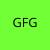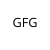Below is the implementation:

## Python3

 `# Importing arcade module``import` `arcade` `# Creating MainGame class``class` `MainGame(arcade.Window):``    ``def` `__init__(``self``):``        ``super``().__init__(``600``, ``600``, title``=``"Player Movement"``)` `        ``# Initializing a variable to store``        ``# the velocity of Player1 and Player2``        ``self``.vel_x1 ``=` `380``        ``self``.vel_x2 ``=` `380` `        ``self``.scene ``=` `None` `        ``# Creating variable to store player sprite``        ``self``.player1 ``=` `None` `        ``# Creating variable to store player sprite``        ``self``.player2 ``=` `None` `    ``# Creating on_draw() function to draw on the screen``    ``def` `on_draw(``self``):``        ``arcade.start_render()` `        ``# Drawing the scene``        ``self``.scene.draw()` `    ``def` `setup(``self``):` `        ``# Creating scene``        ``self``.scene ``=` `arcade.Scene()` `        ``# Adding player sprite``        ``self``.player_sprite1 ``=` `arcade.Sprite(``"Player.png"``, ``1``)``        ``self``.player_sprite2 ``=` `arcade.Sprite(``"Player2.png"``, ``1``)` `        ``# Adding coordinates for the center of the sprite``        ``self``.player_sprite1.center_x ``=` `20``        ``self``.player_sprite1.center_y ``=` `300` `        ``# Adding coordinates for the center of the sprite``        ``self``.player_sprite2.center_x ``=` `580``        ``self``.player_sprite2.center_y ``=` `300` `        ``# Adding sprites in scene``        ``self``.scene.add_sprite(``'Player'``, ``self``.player_sprite1)``        ``self``.scene.add_sprite(``'Player'``, ``self``.player_sprite2)` `    ``# Creating on_update function to``    ``# update the x coordinate``    ``def` `on_update(``self``, delta_time):` `        ``# Changing x coordinate of players``        ``self``.player_sprite1.center_x ``+``=` `self``.vel_x1 ``*` `delta_time``        ``self``.player_sprite2.center_x ``-``=` `self``.vel_x2 ``*` `delta_time` `        ``# Checking if sprites are colliding or not``        ``colliding ``=` `arcade.check_for_collision(``            ``self``.player_sprite1, ``self``.player_sprite2)` `        ``# If sprites are colliding then changing direction``        ``if` `colliding:``            ``self``.vel_x1 ``*``=` `-``1``            ``self``.vel_x2 ``*``=` `-``1` `        ``# Changing the direction if sprites crosses the screen boundary``        ``if` `self``.player_sprite1.center_x > ``600` `or` `self``.player_sprite1.center_x < ``0``:``            ``self``.vel_x1 ``*``=` `-``1` `        ``if` `self``.player_sprite2.center_x > ``600` `or` `self``.player_sprite2.center_x < ``0``:``            ``self``.vel_x2 ``*``=` `-``1`  `# Calling MainGame class``game ``=` `MainGame()``game.setup()``arcade.run()`

Output: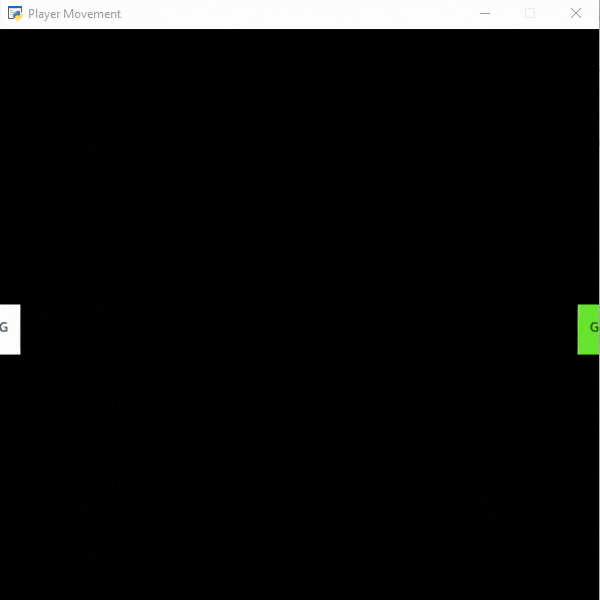## Example 2:

In this example, we are going to use PhysicsEnginePlatformer() function to add collision between our player and platform.

Parameters:

• player_sprite:- The player sprite
• platforms:- The platform sprite list
• gravity:- Amount of gravity

#### Sprites Used: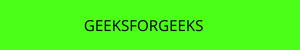Below is the implementation:

## Python3

 `# Importing arcade module``import` `arcade` `# Creating MainGame class``class` `MainGame(arcade.Window):``    ``def` `__init__(``self``):``        ``super``().__init__(``600``, ``600``, title``=``"Player Movement"``)` `        ``# Initializing a variable to store``        ``# the velocity of the player``        ``self``.vel_x ``=` `0` `        ``# Creating scene object``        ``self``.scene ``=` `None` `        ``# Creating variable to store player sprite``        ``self``.player ``=` `None` `        ``# Creating variable for our game engine``        ``self``.physics_engine ``=` `None` `    ``# Creating on_draw() function to draw on the screen``    ``def` `on_draw(``self``):``        ``arcade.start_render()` `        ``# Drawing our scene``        ``self``.scene.draw()` `    ``def` `setup(``self``):``      ` `        ``# Initialize Scene object``        ``self``.scene ``=` `arcade.Scene()` `        ``# Creating different sprite lists``        ``self``.scene.add_sprite_list(``"Player"``)``        ``self``.scene.add_sprite_list(``"Platforms"``, use_spatial_hash``=``True``)` `        ``# Adding player sprite``        ``self``.player_sprite ``=` `arcade.Sprite(``"Player.png"``, ``1``)` `        ``# Adding coordinates for the center of the sprite``        ``self``.player_sprite.center_x ``=` `64``        ``self``.player_sprite.center_y ``=` `600` `        ``# Adding Sprite in our scene``        ``self``.scene.add_sprite(``"Player"``, ``self``.player_sprite)` `        ``# Adding platform sprite``        ``platform ``=` `arcade.Sprite(``"Platform.png"``, ``1``)``        ` `        ``# Adding coordinates for the center of the platform``        ``platform.center_x ``=` `300``        ``platform.center_y ``=` `32``        ``self``.scene.add_sprite(``"Platforms"``, platform)` `        ``# Creating Physics engine``        ``self``.physics_engine ``=` `arcade.PhysicsEnginePlatformer(``            ``self``.player_sprite, ``self``.scene.get_sprite_list(``"Platforms"``), ``0.5``        ``)` `    ``# Creating on_update function to``    ``# update the x coordinate``    ``def` `on_update(``self``, delta_time):` `        ``# Changing x coordinate of player``        ``self``.player_sprite.center_x ``+``=` `self``.vel_x ``*` `delta_time``        ` `        ``# Updating the physics engine to move the player``        ``self``.physics_engine.update()` `    ``# Creating function to change the velocity``    ``# when button is pressed``    ``def` `on_key_press(``self``, symbol, modifier):` `        ``# Checking the button pressed``        ``# and changing the value of velocity``        ``if` `symbol ``=``=` `arcade.key.LEFT:``            ``self``.vel_x ``=` `-``300``        ``elif` `symbol ``=``=` `arcade.key.RIGHT:``            ``self``.vel_x ``=` `300` `    ``# Creating function to change the velocity``    ``# when button is released``    ``def` `on_key_release(``self``, symbol, modifier):` `        ``# Checking the button released``        ``# and changing the value of velocity``        ``if` `symbol ``=``=` `arcade.key.LEFT:``            ``self``.vel_x ``=` `0``        ``elif` `symbol ``=``=` `arcade.key.RIGHT:``            ``self``.vel_x ``=` `0` `# Calling MainGame class``game ``=` `MainGame()``game.setup()``arcade.run()`

Output: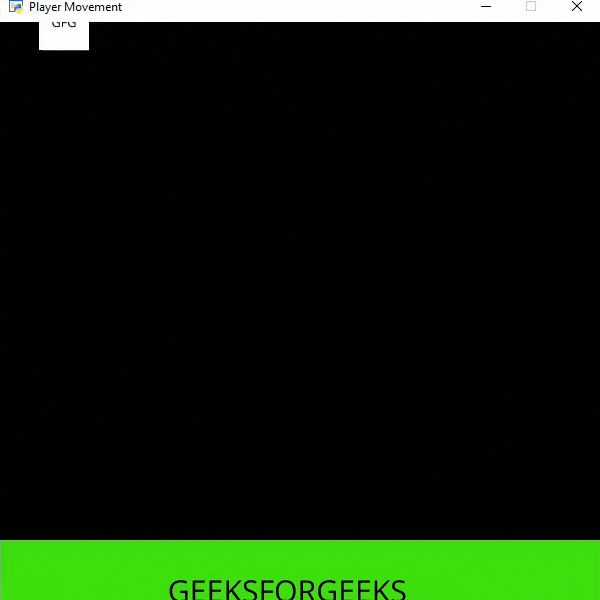My Personal Notes arrow_drop_up